# Calculation Strategies

The Calculation Strategies workbook set teaches the fundamental calculation strategies that your child needs to know: 'Make 10 and Then', 'Near Number Bonds/Doubles', and 'Adjusting from 10'.

Flashcards, mats and counters are included to make the learning practical, visual and fun. Guidance for tutors and parents on how to support and maximise their child's learning is provided throughout the workbooks, including on how to use the animations in the Home Learning Zone to master key concepts and to gently check progress.

## Workbooks

1. Make 10 and Then

Age 6+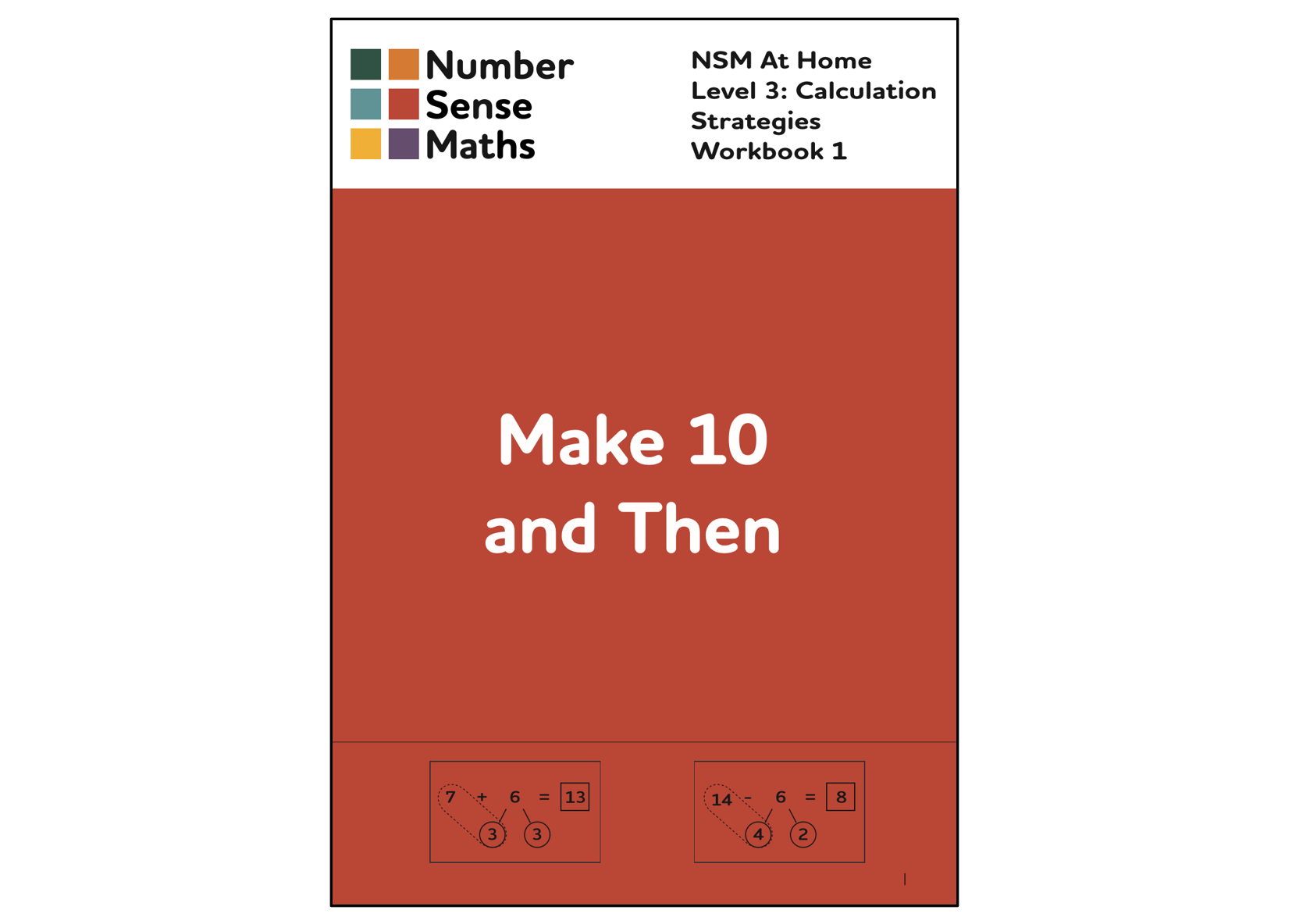31 pages

The 'Make 10 and Then' strategy enables efficient addition and subtraction across 10, for example, calculating 8 + 7, or 13 - 8. It converts a difficult one step equation into an easier two step equation. For example, 8 + 7 becomes 8 + 2 + 5, and 13 - 8 becomes 13 - 3 - 5. The strategy requires good knowledge of subitising, of partitioning (making and breaking), and of number bonds to 10. These skills and facts are taught in earlier Number Sense Maths At Home workbooks.

This workbook first teaches the 'Make 10 and Then' strategy for addition, and then moves on to subtraction. As well as activities and exercises, the book uses animations which explain the strategy visually to parents, and animations which parents and children watch together. Mastering this calculation approach is critical before moving on to column addition and subtraction, where calculations such as these need to be performed in quick succession within one sum.

2. Near Bonds/Doubles

Age 6+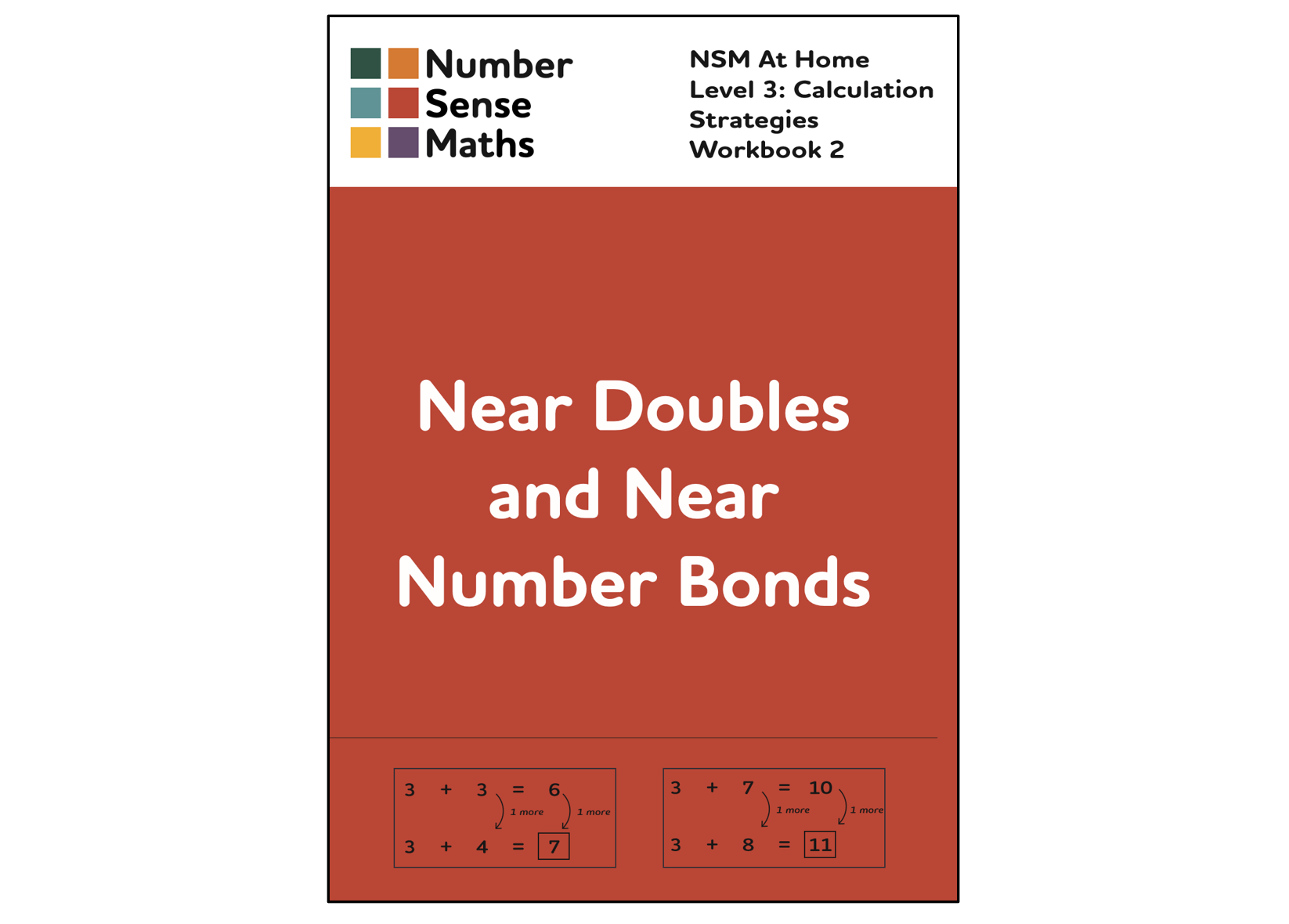27 pages

Near Number Bonds to 10 and Near Doubles are facts that are one more or one less than a number bond to 10 or a double fact. For example, 4 + 7 is a near number bond to 10 since it is one more than 4 + 6, and 7 + 8 is a near double since it is one more than double 7 (or one less than double 8).

When bonds to 10 and doubles are known (they are taught visually and practically in earlier workbooks) we can learn to recall and adjust these facts to solve equations that are close to them but that we don't yet know the answer to.

This workbook teaches this approach. It teaches children to spot equations that they can solve by recalling and adjusting a known fact. Not only does this make it more efficient to solve these equations, it also teaches the fundamental approach to 'use what you know to work out what you don't yet know'. It teaches children to look out for number patterns and number relationships to simplify any equations and problems they face.

Age 6+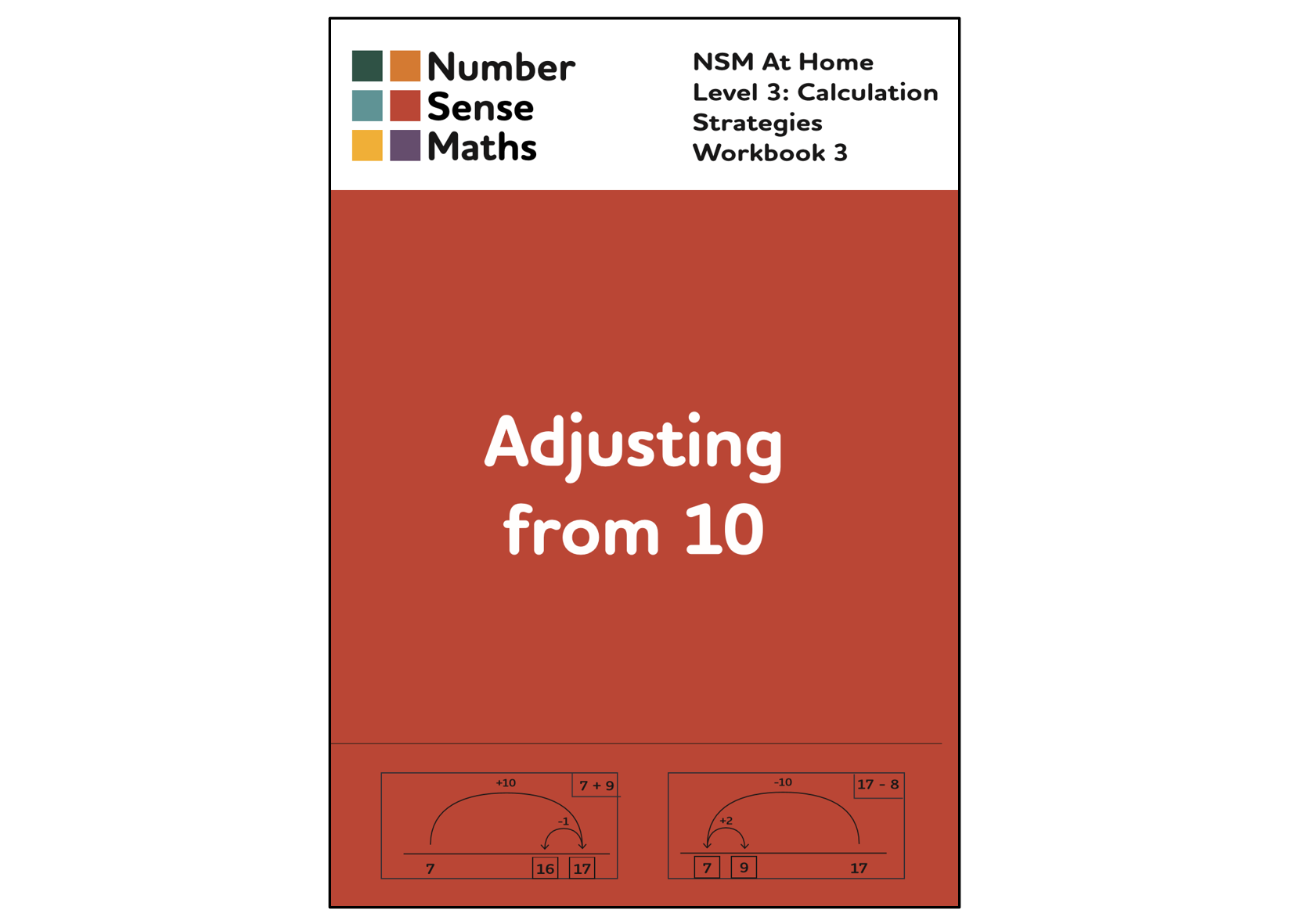27 pages

'Adjusting from 10' provides an efficient way to add or subtract 9 or 8. For example, to solve 7 + 9 using ‘Adjusting from 10’, we calculate 7 + 10 and then we take away 1. Using this approach further develops children's ability to ‘use what they know to work out what they don’t yet know’, and to look for ways to simplify the maths problems that they face.

This workbook teaches children how to use the ‘Adjusting from 10’ strategy and how to spot when to use it. To use the strategy your child needs to be confident adding and subtracting 10. This is taught visually and practically in the earlier Fundamentals workbook ‘Ten and A Bit’.

Strategy selection exercises at the end of the book pull together the three strategies taught in the Calculation Workbooks. These exercises teach children how to choose a strategy when faced with a range of options. With this in place they can solve addition and subtraction equations with confidence and will be looking out for number patterns and relationships to help them in maths.

## Also Included

Flashcards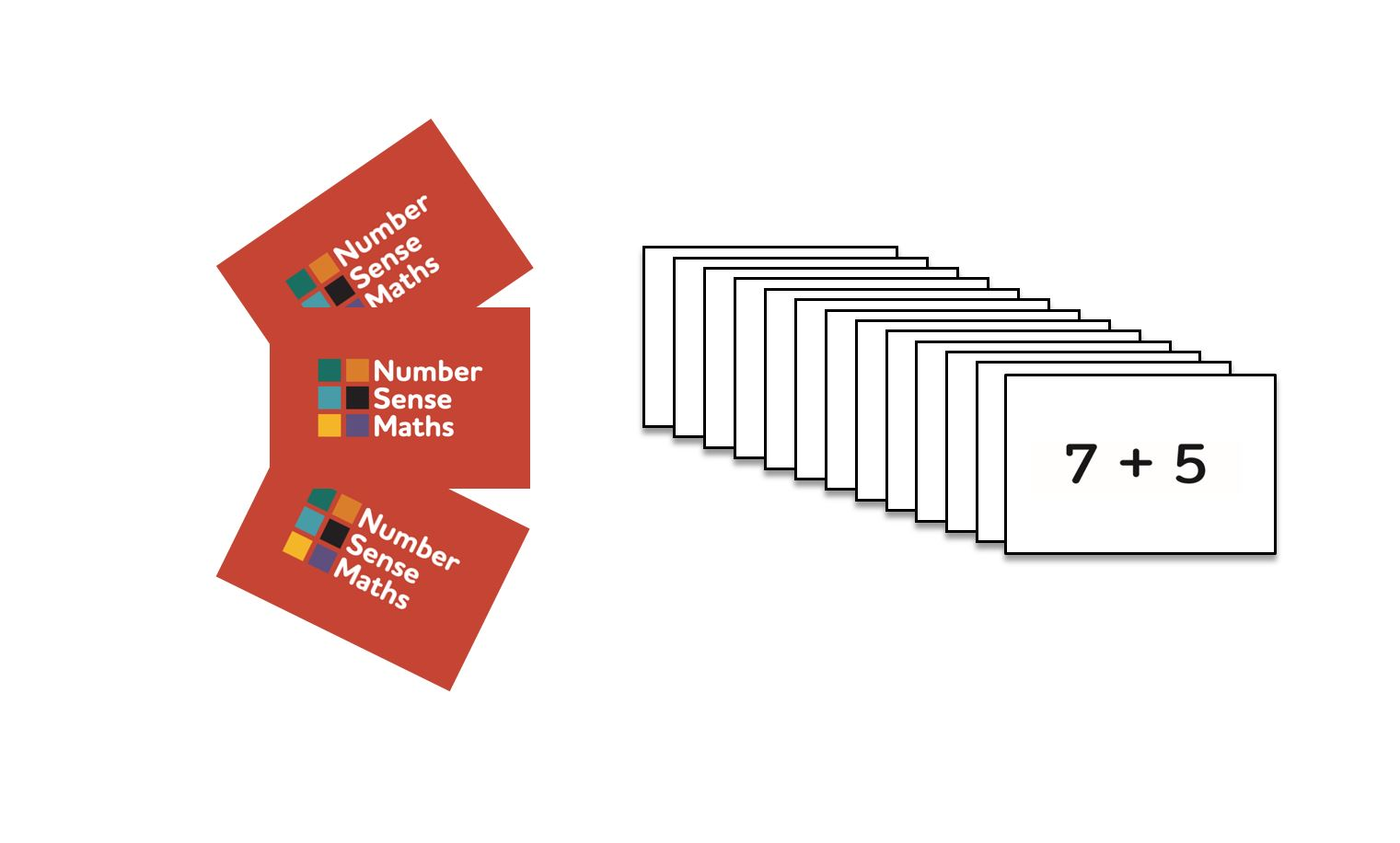A set of 72 flashcards containing the core addition and subtraction facts across 10 that your child needs to be able to solve efficiently by calculation. Use them to check progress along the way, and then little and often on completion of the workbook to keep up your child's confidence with the strategies.

Resource Pack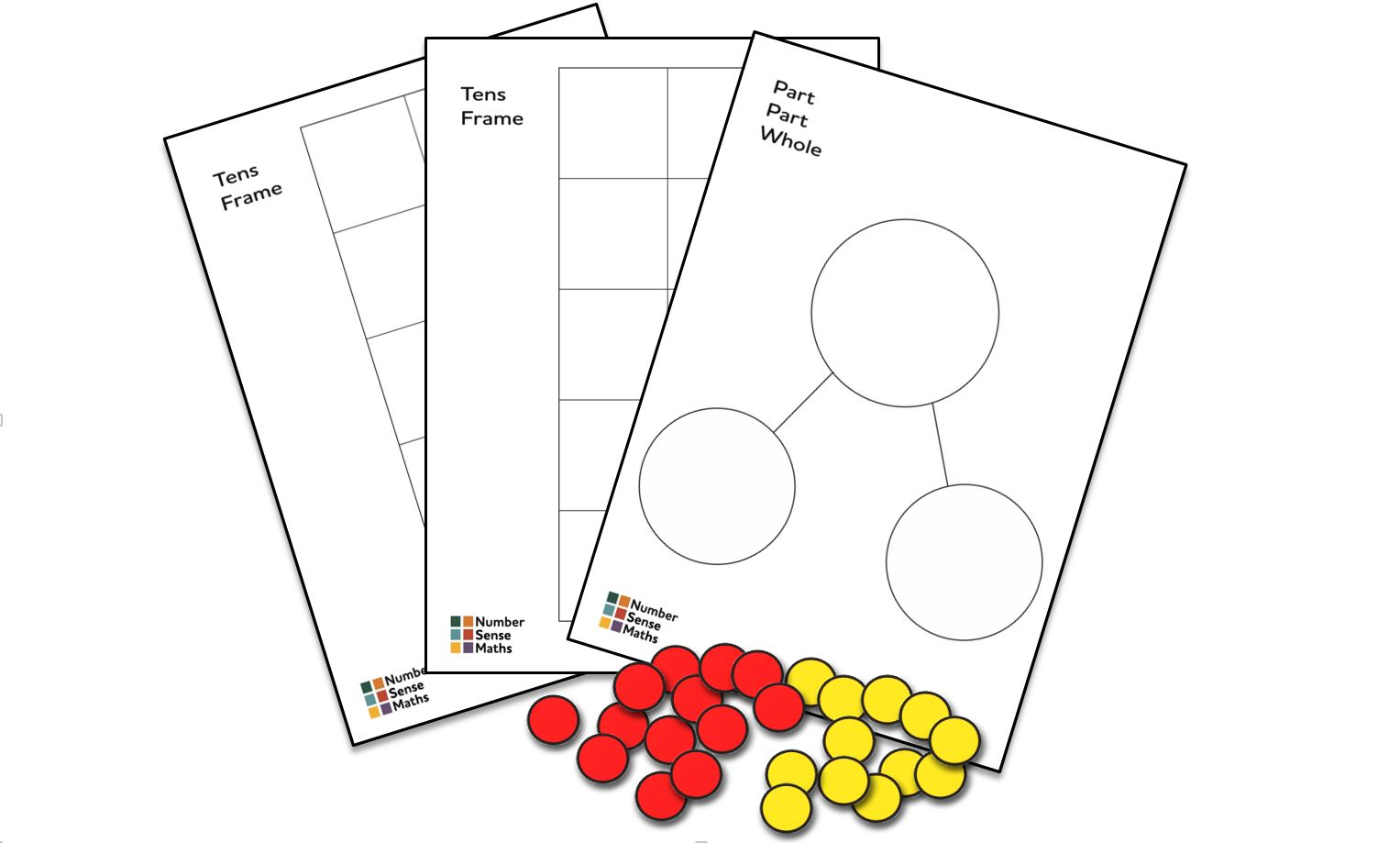Practical and visual resources that will help your child to learn and practise the calculation strategies. Includes a blank A4 part part whole model, two blank A4 tens frame grids, and 25 double sided counters. Use to complete the activities and exercises in the workbooks.